### Showing:

 Annotations Attributes Diagrams Properties Source Used by
Element gml:AffinePlacementType / gml:location
Namespace http://www.opengis.net/gml
Annotations
 ```The location property gives the target of the parameter space origin. This is the vector (x0, y0, z0) in the formulae above.```
Diagram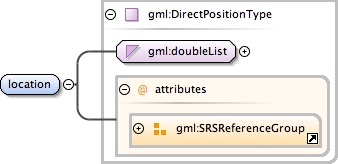Type gml:DirectPositionType
Type hierarchy
Properties
 content: complex
Attributes
QName Type Fixed Default Use Annotation
axisLabels gml:NCNameList optional
 ```Ordered list of labels for all the axes of this CRS. The gml:axisAbbrev value should be used for these axis labels, after spaces and forbiddden characters are removed. When the srsName attribute is included, this attribute is optional. When the srsName attribute is omitted, this attribute shall also be omitted.```
srsDimension positiveInteger optional
 ```The "srsDimension" is the length of coordinate sequence (the number of entries in the list). This dimension is specified by the coordinate reference system. When the srsName attribute is omitted, this attribute shall be omitted.```
srsName anyURI optional
 ```In general this reference points to a CRS instance of gml:CoordinateReferenceSystemType (see coordinateReferenceSystems.xsd). For well known references it is not required that the CRS description exists at the location the URI points to. If no srsName attribute is given, the CRS must be specified as part of the larger context this geometry element is part of, e.g. a geometric element like point, curve, etc. It is expected that this attribute will be specified at the direct position level only in rare cases.```
uomLabels gml:NCNameList optional
 ```Ordered list of unit of measure (uom) labels for all the axes of this CRS. The value of the string in the gml:catalogSymbol should be used for this uom labels, after spaces and forbiddden characters are removed. When the axisLabels attribute is included, this attribute shall also be included. When the axisLabels attribute is omitted, this attribute shall also be omitted.```
Source
 ``` The location property gives the target of the parameter space origin. This is the vector (x0, y0, z0) in the formulae above. ```
Schema location http://schemas.opengis.net/gml/3.1.1/base/geometryPrimitives.xsd
Element gml:AffinePlacementType / gml:refDirection
Namespace http://www.opengis.net/gml
Annotations
 ```The attribute refDirection gives the target directions for the co-ordinate basis vectors of the parameter space. These are the columns of the matrix in the formulae given above. The number of directions given shall be inDimension. The dimension of the directions shall be outDimension.```
Diagram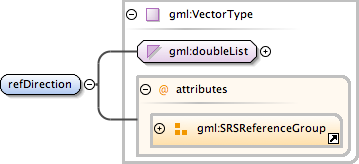Type gml:VectorType
Type hierarchy
Properties
 content: complex maxOccurs: unbounded
Attributes
QName Type Fixed Default Use Annotation
axisLabels gml:NCNameList optional
 ```Ordered list of labels for all the axes of this CRS. The gml:axisAbbrev value should be used for these axis labels, after spaces and forbiddden characters are removed. When the srsName attribute is included, this attribute is optional. When the srsName attribute is omitted, this attribute shall also be omitted.```
srsDimension positiveInteger optional
 ```The "srsDimension" is the length of coordinate sequence (the number of entries in the list). This dimension is specified by the coordinate reference system. When the srsName attribute is omitted, this attribute shall be omitted.```
srsName anyURI optional
 ```In general this reference points to a CRS instance of gml:CoordinateReferenceSystemType (see coordinateReferenceSystems.xsd). For well known references it is not required that the CRS description exists at the location the URI points to. If no srsName attribute is given, the CRS must be specified as part of the larger context this geometry element is part of, e.g. a geometric element like point, curve, etc. It is expected that this attribute will be specified at the direct position level only in rare cases.```
uomLabels gml:NCNameList optional
 ```Ordered list of unit of measure (uom) labels for all the axes of this CRS. The value of the string in the gml:catalogSymbol should be used for this uom labels, after spaces and forbiddden characters are removed. When the axisLabels attribute is included, this attribute shall also be included. When the axisLabels attribute is omitted, this attribute shall also be omitted.```
Source
 ``` The attribute refDirection gives the target directions for the co-ordinate basis vectors of the parameter space. These are the columns of the matrix in the formulae given above. The number of directions given shall be inDimension. The dimension of the directions shall be outDimension. ```
Schema location http://schemas.opengis.net/gml/3.1.1/base/geometryPrimitives.xsd
Element gml:AffinePlacementType / gml:inDimension
Namespace http://www.opengis.net/gml
Annotations
 ```Dimension of the constructive parameter space.```
Diagram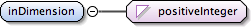Type positiveInteger
Properties
 content: simple
Source
 ``` Dimension of the constructive parameter space. ```
Schema location http://schemas.opengis.net/gml/3.1.1/base/geometryPrimitives.xsd
Element gml:AffinePlacementType / gml:outDimension
Namespace http://www.opengis.net/gml
Annotations
 `Dimension of the co-ordinate space.`
Diagram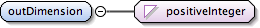Type positiveInteger
Properties
 content: simple
Source
 ``` Dimension of the co-ordinate space. ```
Schema location http://schemas.opengis.net/gml/3.1.1/base/geometryPrimitives.xsd
Complex Type gml:AffinePlacementType
Namespace http://www.opengis.net/gml
Annotations
 ```A placement takes a standard geometric construction and places it in geographic space. It defines a transformation from a constructive parameter space to the co-ordinate space of the co-ordinate reference system being used. Parameter spaces in formulae in this International Standard are given as (u, v) in 2D and(u, v, w) in 3D. Co-ordinate reference systems positions are given in formulae, in this International Standard, by either (x, y) in 2D, or (x, y, z) in 3D. Affine placements are defined by linear transformations from parameter space to the target co-ordiante space. 2-dimensional Cartesian parameter space,(u,v) transforms into 3-dimensional co- ordinate reference systems,(x,y,z) by using an affine transformation,(u,v)->(x,y,z) which is defined : x ux vx x0 u y = uy vy + y0 v x uz vz z0 Then, given this equation, the location element of the AffinePlacement is the direct position (x0, y0, z0), which is the target position of the origin in (u, v). The two reference directions (ux, uy, uz) and (vx, vy, vz) are the target directions of the unit vectors at the origin in (u, v).```
Diagram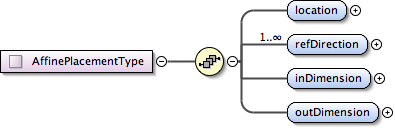Used by
 Element gml:AffinePlacement
Model gml:location , gml:refDirection+ , gml:inDimension , gml:outDimension
Children gml:inDimension, gml:location, gml:outDimension, gml:refDirection
Source
 ``` A placement takes a standard geometric construction and places it in geographic space. It defines a transformation from a constructive parameter space to the co-ordinate space of the co-ordinate reference system being used. Parameter spaces in formulae in this International Standard are given as (u, v) in 2D and(u, v, w) in 3D. Co-ordinate reference systems positions are given in formulae, in this International Standard, by either (x, y) in 2D, or (x, y, z) in 3D. Affine placements are defined by linear transformations from parameter space to the target co-ordiante space. 2-dimensional Cartesian parameter space,(u,v) transforms into 3-dimensional co- ordinate reference systems,(x,y,z) by using an affine transformation,(u,v)->(x,y,z) which is defined : x ux vx x0 u y = uy vy + y0 v x uz vz z0 Then, given this equation, the location element of the AffinePlacement is the direct position (x0, y0, z0), which is the target position of the origin in (u, v). The two reference directions (ux, uy, uz) and (vx, vy, vz) are the target directions of the unit vectors at the origin in (u, v). The location property gives the target of the parameter space origin. This is the vector (x0, y0, z0) in the formulae above. The attribute refDirection gives the target directions for the co-ordinate basis vectors of the parameter space. These are the columns of the matrix in the formulae given above. The number of directions given shall be inDimension. The dimension of the directions shall be outDimension. Dimension of the constructive parameter space. Dimension of the co-ordinate space. ```
Schema location http://schemas.opengis.net/gml/3.1.1/base/geometryPrimitives.xsd